# Working with Permutations in Wolfram|Alpha

November 29, 2011 —Comments Off

Permutations are among the most basic elements of discrete mathematics. They are used to represent discrete groups of transformations, and in particular play a key role in group theory, the mathematical study of symmetry. Permutations and groups are important in many aspects of life. We all live on a giant sphere (the Earth) whose rotations are described by the group SO(3) (the special orthogonal group in 3 dimensions). On the micro-scale, the Hungarian-American physicist Eugene Wigner (November 17, 1902–January 1, 1995), who received a share of the Nobel Prize in Physics in 1963, discovered the “electron permutation group”, one of many applications of permutation groups to quantum mechanics.

Permutations deserve a full treatment in Wolfram|Alpha. Since Mathematica 8 provides new functionality to work with permutations using both list and cyclic representations, we now have a powerful new way of working with permutations using natural language.

Let’s first define permutations, then discuss how to work with them in Wolfram|Alpha. A permutation of a set X is a bijective (one-to-one and onto) mapping of X to itself. There is a convenient way of specifying a permutation α of a finite set of n elements: write down the numbers 1, 2, …, n in a row and write down their images under α in a row beneath: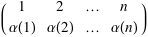We call this two-line notation. For example, the permutation α of {1, 2, 3, 4, 5} with α(1)=3, α(2)=1, α(3)=5, α(4)=2, and α(5)=4 is written: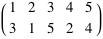Every permutation can be written as a cycle or as a product of disjoint cycles, for example in the above permutation {1 → 3, 3 → 5, 5 → 4, 4 → 2, 2 → 1}. One of the nicest things about a permutation is its cycle decomposition. Many times the most interesting information about a permutation are the lengths of its disjoint cycles. For instance, the following permutation decomposes into three cycles: one of length = 2 and two of length = 3.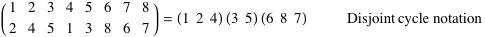Another common notation is a permutation list: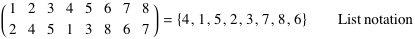Wolfram|Alpha lists these different notations, typically in the first results pod.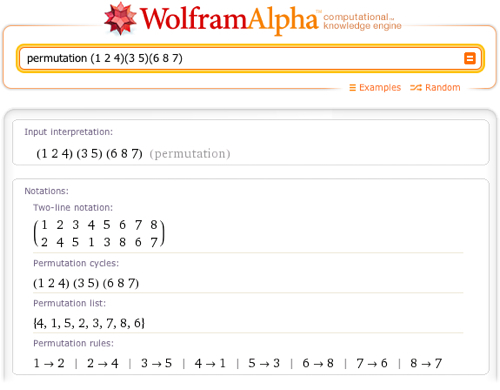We will now compute with permutations on a small set. (Why small? Say there are 100 elements in your set. Then there are 100! permutations, which would take you almost 3 x 10150 years to write if you wrote out one permutation every second.) Let’s start by examining the properties of the permutation (1 2 5 3 7). As you can see, this permutation’s notation pod has a button that toggles the display of fixed points, that is, the numbers that do not move.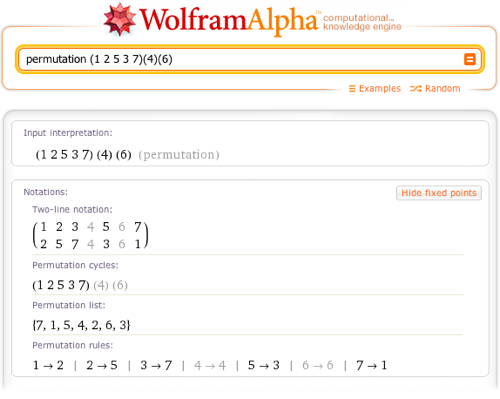A permutation is a bijection, which means that every permutation has an inverse function. Wolfram|Alpha computes a permutation’s inverse and writes it in cycle notation. Then it gives the order of the permutation (when written in disjoint cycle form, the order is simply the least common multiple of the length of the cycles). After that is the index of a permutation α, which is the sum of all subscripts j such that α(j) > α(j + 1), 1 ≤ j < n. If you forget what these terms mean, there is a convenient Definitions button in the lower right corner of the pod, which opens a popup box displaying the definitions of the terms in the pod. Pressing the More button opens up a host of additional properties: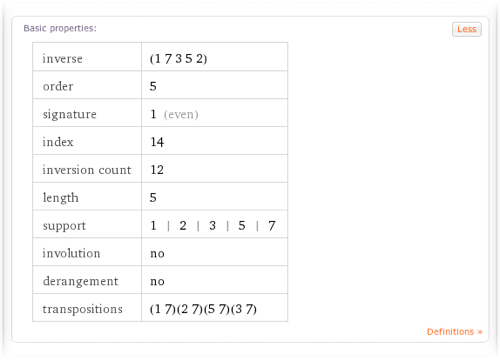If you know which property you’re looking for, just ask Wolfram|Alpha to return that property. For instance, the signature of a permutation is +1 if the number of transpositions (two element cycles) is even and -1 if it is odd. Let’s find the signature or sign of the permutation list {4, 1, 5, 2, 3, 7, 8, 6}.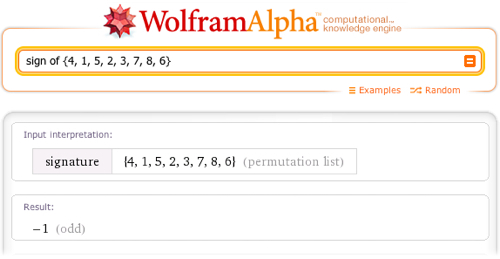Now, function composition is a useful way to write permutations and is used specifically to study powers and products of permutations (note that the order in which you compose permutations matters), and for undergraduates learning about permutations for the first time, Wolfram|Alpha streamlines the learning process.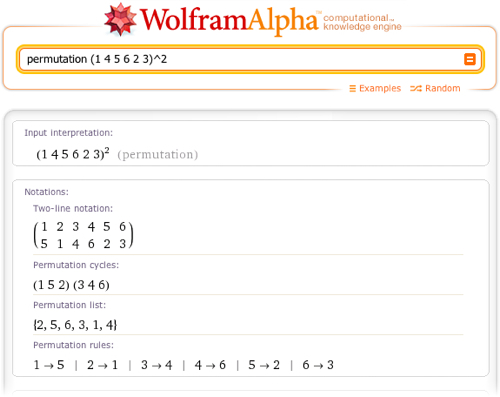With products of permutations, the composition is assumed to be left to right.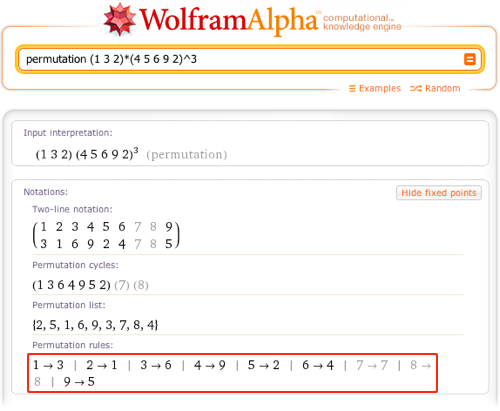Wolfram|Alpha provides a number of graphics to help us better understand permutations. One is the Permutation matrix, which Wolfram|Alpha displays both graphically and numerically: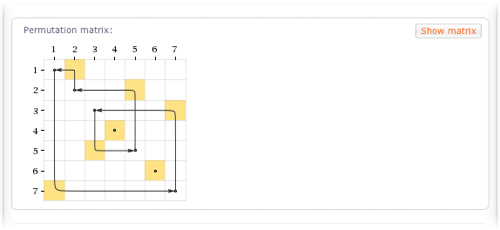The Cycle structure of the permutation nicely exhibits its action: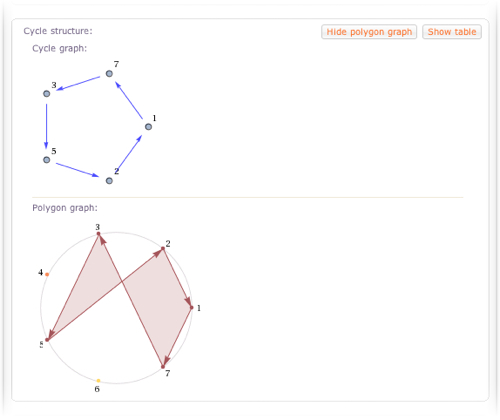Wolfram|Alpha also knows about all kinds of special classes of permutations, such as involutions and derangements: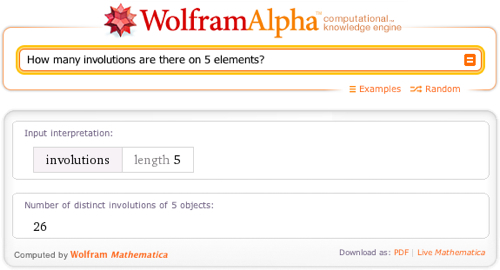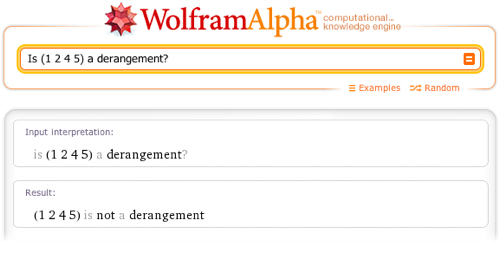In combinatorics, it’s often useful to construct all permutations. Usually the best way of doing this is in lexicographic order. Wolfram|Alpha understands this ordering and can rank and un-rank permutations lexicographically and give a mixed radix numbering for permutations using the factorial number system (also called factoradic).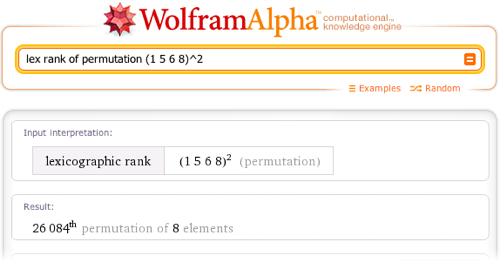Inversion is an important concept in the study of permutations. Inversions are pairs of elements that are out of order in the list form of a permutation. Inversions play a significant role in the analysis of sorting algorithms. One method of highlighting the inversions within a given permutation uses a so-called inversion graph (sometimes called a permutation graph). The inversion graph of a permutation α is a graph whose vertex set is {1, 2, 3, …, n} and whose edges {i, j} correspond exactly to (i, j) being an inversion in α. A clique in the graph corresponds to a decreasing sequence in the corresponding permutation.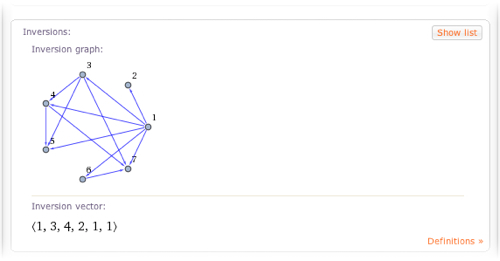Wolfram|Alpha also returns random permutations of any length: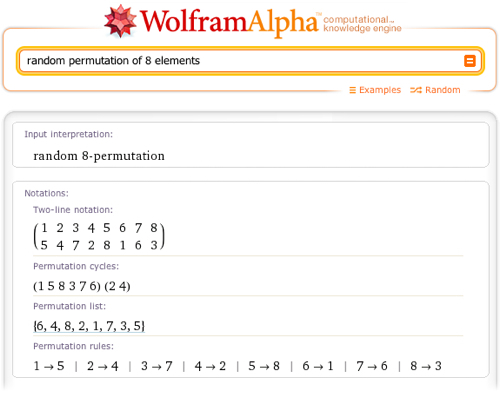Mathematica takes a unified approach to programming, so that new permutation functions seamlessly interact with all other Mathematica expressions. We illustrate this using Wolfram|Alpha: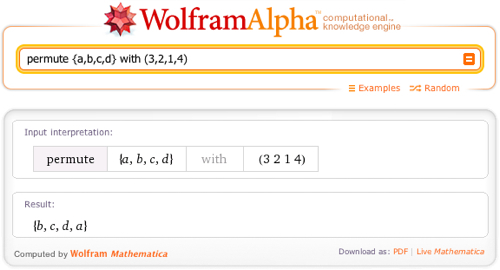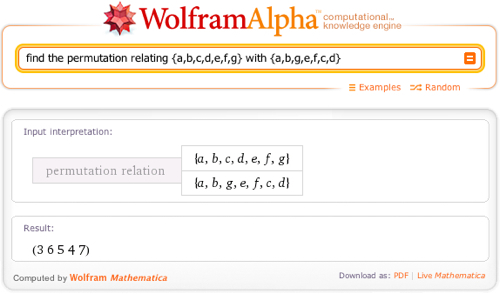There are plenty more applications and use-cases of permutations now in Wolfram|Alpha. Try them out and have fun with them. Also, be on the lookout for a permutation group blog post in the coming weeks!

Excellent, this permutation feature will open many new possibilities.

Posted by P. Seeger November 29, 2011 at 5:13 pm

Thanks Wolfram Alpha 🙂

I’m taking Abstract Algebra 1 and I’m not very computer savy, now I can finally compute powers, products, and inverses really easily!

Posted by Mac Heidt November 30, 2011 at 11:04 pm

Great job you have done. I would recommend you to expand the permutation functionality of using Wolfram Alpha with permutohedron graphs, lehmer code, permutations ordered by the subset relation
between their inversion sets and link with permutation groups.

Posted by B. Radovanovic December 8, 2011 at 2:34 am

Is it just me or does this no longer work? I have tried the exact same input as shown in these images and my results are complete nonsense. Every composition of permutations is the identity permutation according to wolframalpha…

Posted by mike September 19, 2012 at 7:19 pm

nevermind apparently I forgot the spaces. BAH

Posted by mike September 19, 2012 at 7:20 pm

Ok actual comment this time. You say that “With products of permutations, the composition is assumed to be left to right.” Why is that? Most books I’ve seen and classes I’ve taken have the composition going right to left just like function composition.

Posted by mike September 19, 2012 at 7:22 pm

Can anyone solve this problem form me?
1) How many five-letter codes can be form using the letters in the word ’WINSDOM’ such that the vowels are place adjacent to each others
2) How many seven-letter codes can be form using the letters in the word ’SINGAPORE’ such that the vowels are place adjacent to each others
3) How many seven–letter codes can be form using the letters in the word ’SINGAPORE’ such that the vowels are place separately to each others.
4) How many five-letter codes can be form using the letters in the word ’COCONUT’ such that the vowels are place adjacent to each others?

Posted by Terance May 19, 2014 at 9:12 pm

How do I display all combinations and permutations of, for instance, three numbers (1,2,3) but with a resulting length of four numbers? I.e. including repeating numbers, but all three must exist in all combinations.
I.e. (1,2,2,3) and (1,1,2,3) is correct but (1,1,3,3) is not because there is no 2 included.

Posted by Daniel November 13, 2014 at 6:40 pm

Thank you for your comment, unfortunately Wolfram|Alpha does not have that capability.

Posted by The Wolfram Team November 17, 2014 at 11:58 am

How do i type out a 2 line permutation

Posted by ansar September 27, 2015 at 12:07 am